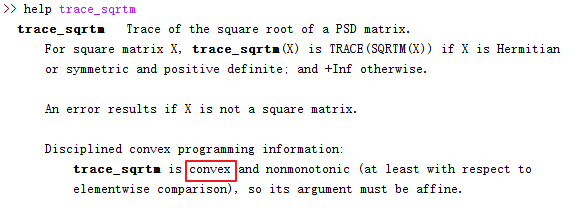# Documentation error regarding the "trace_sqrtm" function in the Matlab CVX 2.2

There is some documentation errors regarding the “trace_sqrtm” function in the Matlab CVX 2.2 .

Actually, “trace_sqrtm” is concave. Thus, it should be replace the “convex” with the “concave” in the trace_sqrtm matlab help information.CVX: Software for Disciplined Convex Programming ©2014 CVX Research
Version 2.2, Build 1148 (62bfcca) Tue Jan 28 00:51:35 2020

You are correct. The help is incorrect.

However, the CVX Users’ Guide is correct in this case:

trace_sqrtm(X) trace of the matrix square root of a positive semidefinite matrix X. which is the same as the sum of the squareroots of the eigenvalues. Concave. Outside of CVX, returns +Inf if argument is not positive semidefinite.

With such attentive reading as you have exhibited in discovering two CVX documentation errors, you should be in good shape for using CVX. You are hereby awarded a free virtual beer